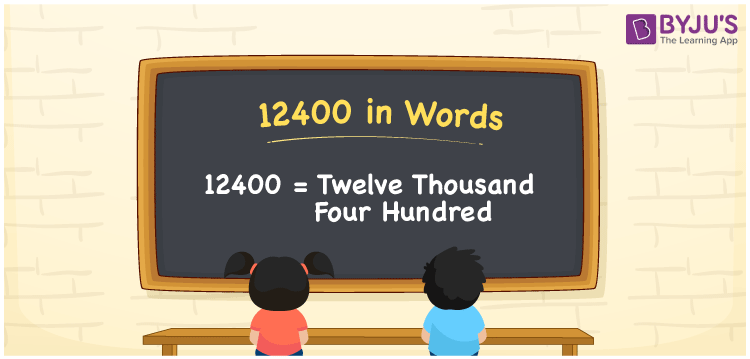# 12400 in Words

We can write 12400 in words as Twelve thousand four hundred. As we know, 12400 is a cardinal number because it illustrates a certain quantity. If you paid Rs. 12400 to buy a new inverter, you could say, “I purchased an inverter worth Twelve thousand four hundred rupees”.

 12400 in words Twelve thousand four hundred Twelve thousand four hundred in numerical form 12400

## 12400 in English Words

Generally, we use English alphabets to write numbers in words, and so, we can spell 12400 in English words as “Twelve thousand four hundred”.## How to Write 12400 in Words?

In this section, you will learn how to write the number 12400 in words using a five-column place value table as given below.

 Ten thousands Thousands Hundreds Tens Ones 1 2 4 0 0

Here, we can observe that ones = 0, tens = 0, hundreds = 4, thousands = 2, ten thousands = 1

So, 1 × Ten thousand + 2 × Thousand + 4 × Hundred + 0 × Ten + 0 × One

= 1 × 10000 + 2 × 1000 + 4 × 100 + 0 × 10 + 0 × 1

= 10000 + 2000 + 400

= Ten Thousand + Two thousand + Four hundred

= Twelve thousand + four hundred

= Twelve thousand four hundred

12400 is a natural number that is the successor of 12399 and predecessor of 12401.

12400 in words – Twelve thousand four hundred

Is 12400 an odd number? – No

Is 12400 an even number? – Yes

Is 12400 a prime number? – No

Is 12400 a composite number? – Yes

Is 12400 a perfect square number? – No

Is 12400 a perfect cube number? – No

## Frequently Asked Questions on 12400 in Words

Q1

### How do you write 12400 in words?

12400 can be written in words as Twelve thousand four hundred.
Q2

### How do you write Rupees 12400 on a cheque?

On a cheque, Rs. 12400 can be written as “Twelve thousand four hundred rupees only”.
Q3

### Write the value of 8400 + 4000 in words.

8400 + 4000 = 12400 The value of 8400 + 4000, i.e. 12400 in words is Twelve thousand four hundred.
Test your Knowledge on 12400 in Words